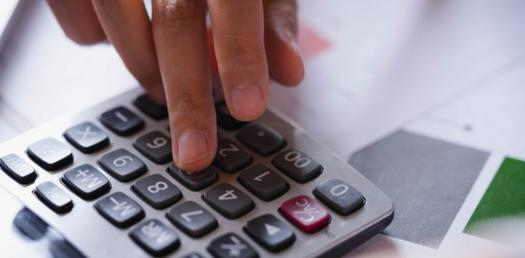# Quiz: Business Mathematics & Statistics #6

20 Questions | Attempts: 172
ShareSettings• 1.
A, B and C play cricket. A's runs are to B's runs and B's runs are to C's as 3:2. They get altogether 342 runs. How many runs did A make?
• A.

162

• B.

108

• C.

72

• D.

112

• 2.
The price of a P4 computer was \$12000 last year. What is the price of the computer for this year if the price is decreased by 10%?
• A.

\$12000

• B.

\$12500

• C.

\$10800

• D.

\$9000

• 3.
Four friends take an IQ test. Their scores are 96, 100, 106, 114. Which of the following statements is true?
• A.

The mean is 103.

• B.

The mean is 104.

• C.

The median is 100.

• D.

The median is 106.

• 4.
Percentage is a ratio of given number with standard number:
• A.

100

• B.

Same number

• C.

1000

• D.

10

• 5.
The Virtual University of Pakistan is a growing distant learning university of Pakistan. The number of students in the university is increasing each year. The number of registered students in a course related to business math last year was 7113 and it is increased up to 9242 this year. What is the percentage increase in the number of students?
• A.

23%

• B.

30%

• C.

18%

• D.

42%

• 6.
If A and B are any two matrices of order  and  respectively and m>n and p. What should be the condition on m, n, p, q for the product AB to hold?
• A.

n>p

• B.

m

• C.

Q=p

• D.

N=p

• 7.
The price at which investors buy or sell a share of stock at a given time is referred as
• A.

Face value

• B.

Market value

• C.

Accumulated value

• D.

Earning value

• 8.
Gross salary is also called Net salary
• A.

True

• B.

False

• 9.
Can we subtract a positive integer k from the identity matrix?
• A.

Yes

• B.

No

• 10.
Net price =
• A.

• B.

• C.

• D.

• 11.
Division. Symbol: /  is  a type of
• A.

Comparison operators

• B.

Concatenation operator

• C.

Reference operators

• D.

Arithmetic operators

• 12.
Given :  List Price =5500Rs   , Discount = 850Rs, then  Net Cost Price will be
• A.

6350 Rs

• B.

5508 Rs

• C.

5585 Rs

• D.

4650 Rs

• 13.
To convert a number in percent (%) from a fraction, we do which of the following operation:
• A.

• B.

subtract by 10

• C.

Multiply by 100

• D.

Divide by 100

• 14.
If is the matrix of dimension  and is the identity matrix of dimension . Then which of the following is true
• A.

AI = I

• B.

AI = A

• C.

A + I = A

• D.

A + I = I

• 15.
If ratio of boys and girls in a class is 7: 5, which of the following can’t be the total number of the students in the class?
• A.

36

• B.

50

• C.

60

• D.

120

• 16.
SLN returns the straight-line depreciation of an asset for
• A.

Zero period

• B.

One period

• C.

Two period

• D.

Three periods

• 17.
Assume you will work for the next 20 years and at the end of each year you will deposit \$20,000 into a savings account.  At the end of that 20 year period you will start withdrawing your retirement living expenses from that account at the beginning of every year.  This financial plan involves a _____ problem while you are working and a _____ problem while you are in retirement.
• A.

A present value of a dollar, future value of a dollar

• B.

A future value of an annuity due, a present value of an annuity due

• C.

A future value of an annuity due, a present value of an ordinary annuity

• D.

A future value of an ordinary annuity, a present value of an annuity due

• E.

a future value of an ordinary annuity, a present value of an ordinary annuity

• 18.
which of the following is linear equatio
• A.

2x-3y=-6

• B.

X+x+x

• C.

520x²y²

• D.

Y^2 -3=0

• 19.
Given  FC = Rs.5000 ,CM= Rs. 30 ,VC= Rs. 150 , Capacity = 320units then BEP in units =
• A.

4500 units

• B.

167 units

• C.

33 units

• D.

16 units

• 20.
Find the Discount where price is 2000 and discount rate is 12%  Solution  Price = 2000
• A.

Discount rate = 12%

• B.

Discount = Price * rate

• C.

Discount = 2000*12/100

• D.

Discount = 240

## Related TopicsBack to top
×

Wait!
Here's an interesting quiz for you.